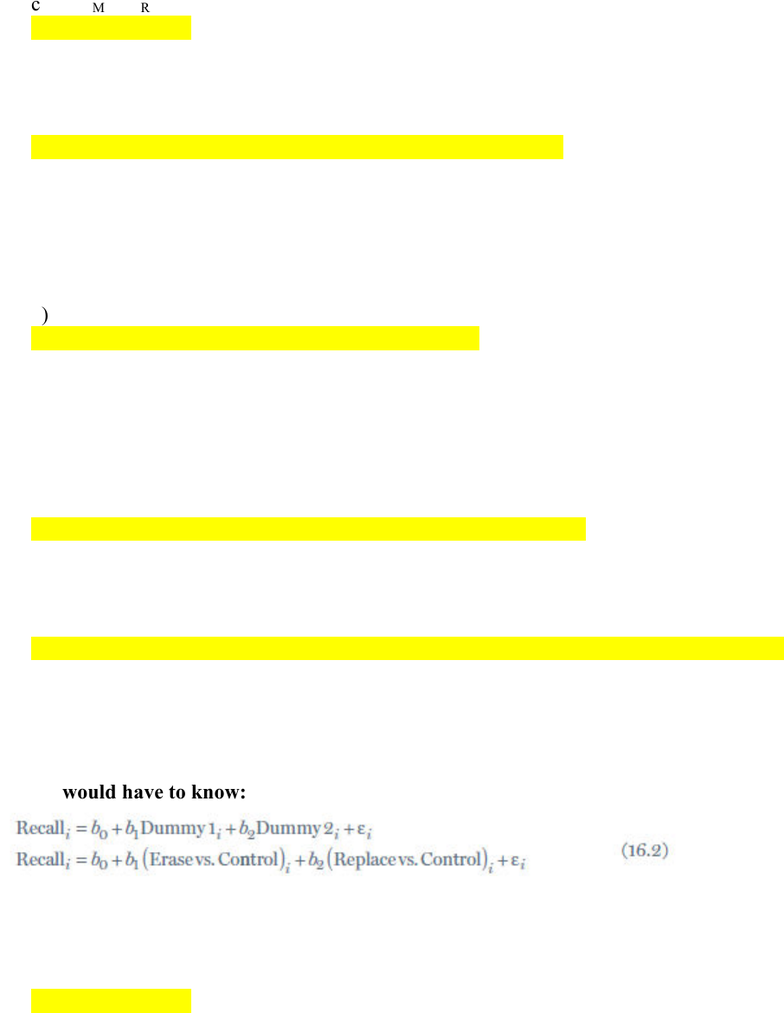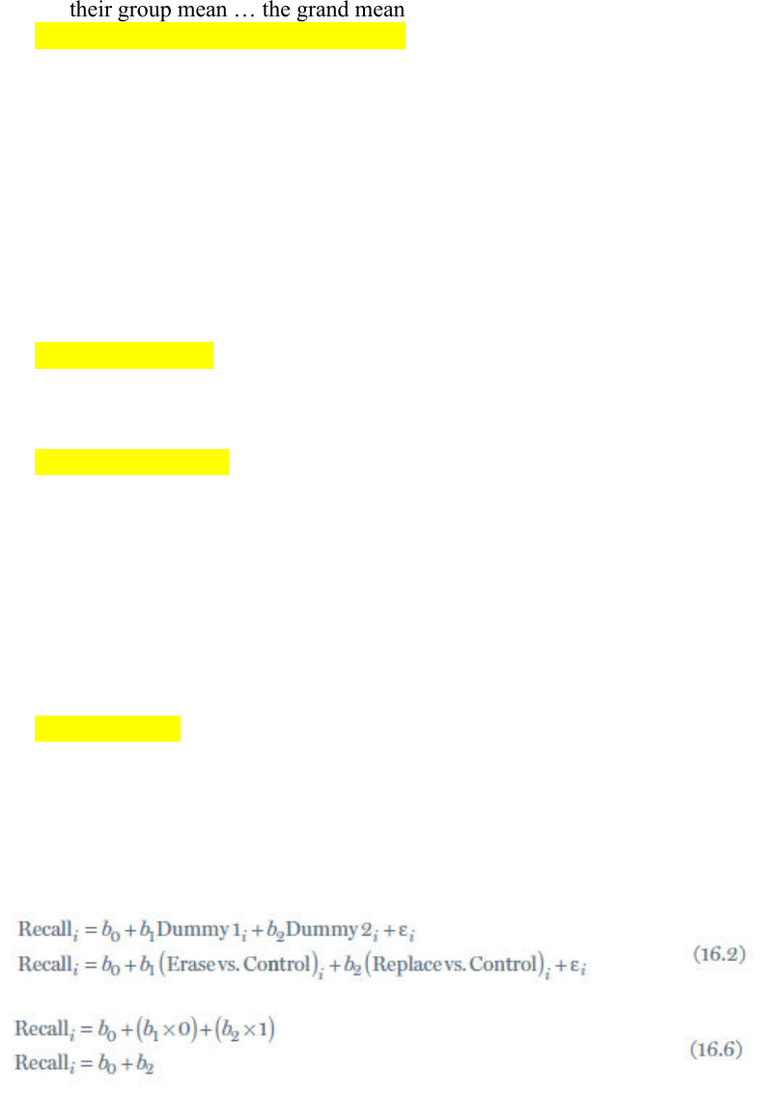# PNB 3XE3 Study Guide - Quiz Guide: Variance Inflation Factor, Exclusive Or, Groupwise

50 views15 pagesChapter 16:
Homework 14
1. The residual degrees of freedom, dfR:
a) is the square root of the sum of degrees of freedom for each group
b) N k
c) n 1
d) none of the above
2. An ANOVA is
a) a special case of a linear model
b) an analysis of variance
c) an analysis that is common in experimental designs in psychology
d) all of the above
3. If you want to compare 4 groups using an ANOVA, how many dummy variables do
you need?
a) 1
b) 2
c) 3
d) 4
4. In figure 16.4 does the horizontal location of a case have any predictive value, as
long as it stays within a group?
a) yes
b) no
c) there is not enough information
5. Before you conduct an ANOVA, you may want to check for equal variance in your
groups using:
a) Cook’s distance
b) Levene’s test
c) Shapiro-Wilk tests
d) the variance inflation factor
Unlock document

This preview shows pages 1-3 of the document.
Unlock all 15 pages and 3 million more documents.6. The F-ratio is:
a a measure of the ration of systematic variation to unsystematic variation
b the ratio of the experimental effect to the individual differences in performance
c MSM/MSR
d all of the above
7. In an ANOVA, b2 equals:
a) the difference between the third group and the second group
b) the difference between the third group and the control group
c) the mean of the third group
d) none of the above
8. The total sum of square is:
a) how much variability the model can not explain
b) how much of the total variability is accounted for by the model
c) how much variability in outcome scores there was
d) none of the above
9. In an ANOVA, b0 = ___, and in an independent t-test, b0 = ___
a) the means of the experimental group … the mean of the control group
b) the slop between the control group and the experimental group … the mean of the control
group
c) the mean of the control group … the mean of the control group
d) none of the above
10. When conducting an analysis of variance, the expected value of the outcome
variable associated with a given case is equal to ___
a) the group mean of the outcome variable of the group that the particular case belongs to
b) the grand mean
c) the average of all group means
d) the residual
11. Given the following equations, in order to predict a person’s “Recall” score, you
would have to know:
a) their group membership
b) the difference between their group’s mean and the control mean (b’s)
c) the intercept
d) all of the above
Unlock document

This preview shows pages 1-3 of the document.
Unlock all 15 pages and 3 million more documents.12. In an ANOVA, is the null hypothesis is true, then the best predictor of a
participant’s score is ___. If the alternative hypothesis is true, the best predictor of
the participant score is ___.
a) their group mean the grand mean
b) the grand mean … their group mean
c) the grand mean … the grand mean
d) none of the above
13. Dummy coding …
a) is a way of recoding a categorical variable with two or more categories into numerical
variables
b) requires 7 steps, although the 6th step is “Repeat step 5 until you run out of dummy
variables”
c) allows you to calculate the parameter for each predictor, which is the difference between
the mean of that condition compared to the mean of the control condition
d) all of the above
14. When using dummy coding, which group is assigned the code “zero”?
a) the control group
b) the smallest group
c) the group that, based on previous research, is expected to predict the outcome most
strongly
d) the group that is of greatest experimental interest
15. When you predict an outcome from one predictor variable, and that variable is
made up of 3 groups, this analysis is called:
a) a t-test
b) an ANOVA
c) a regression analysis
d) a correlation
16. Given the following equations, if you use the second pair of equations below to
calculate a subject’s predicted score:
Unlock document

This preview shows pages 1-3 of the document.
Unlock all 15 pages and 3 million more documents.